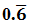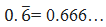September 30, 2023

# Rational Numbers Class 9 Worksheet

If you are a Class 9 student studying rational numbers  and looking for a comprehensive worksheet on Rational Numbers , this worksheet will help you test your knowledge and understanding of the subject. Rational Numbers Class 9 Worksheet with answers will help you  to  improve your understanding of rational numbers.

This worksheet is filled with exercises that will require you to think critically and use your understanding of rational numbers. This worksheet is useful whether you are studying for a test or simply reviewing your understanding of rational numbers.

Whether you’re a student looking to revise your understanding of rational numbers or a teacher looking for an effective resource to help your students master this essential mathematical concept, this rational numbers class 9 worksheet with answers pdf worksheet is the perfect for you. So, get your pencils ready and start practicing rational numbers today!

## Class 9 Rational Numbers Solved Examples

Example 1: Find  6 rational numbers between and

Sol.  To solve this problem we will use GAP Method to find rational numbers between two given numbers.

Let    and , then    . Gap between and     =    .

To find  6 rational numbers , divide by .

Dividing the gap by 7, we get

Thus the 6 rational numbers between   and are

,    ,    , and   ,

i.e. .

Example 2 : Find a rational number between   and  .

Sol. Suppose  and are two rational numbers  such that , then is a rational number lying between  and .

Here and

required rational number between  and   =   =

=

Hence   is a rational number  between   and  .

Example 3: Find six rational number between 4 and 5 .

Sol. To find six rational  numbers between 4 and 5, we will convert given rational numbers into equivalent rational number  by multiplying the numerator and denominator by a suitable number , usually (n+1). Here  n= 6
We will convert  4 and 5 into equivalent rational numbers by multiplying 7  as multiplying factor.
Thus and

Now

or

Hence six rational numbers between 4 and 5 are .

Example 4 : Without actual division , find which of the following is a terminating decimal (i)        (ii)         (iii)
Sol.  A rational number is a terminating decimal only when prime factors of are 2 and 5 only.
(i) Denominator of  is 32  and
32 has no prime factors other than 2.
So,  is a terminating decimal.

(ii) Denominator of  is 8   and
8 has no prime factors other than 2.
So,   is a terminating decimal.

(iii) Denominator of   is 24   and
24 has a  prime factor 3, which is  other than 2 and 5.
So,    is  not a terminating decimal.

Example 5: Write the following in decimal form and say what kind of decimal expansion each has :

(a)
Sol.
By actual division , we have  =  0.2142857142857………….. =
Clearly ,  has a non-terminating recurring decimal representation.

(b)
Sol.
By actual division , we have  =  0.09090909………….. =
Clearly ,  has a non-terminating recurring decimal representation.

(c)
Sol.  By actual division , we have
Clearly ,  has a terminating  decimal representation.

Example 6:  Is zero a rational number? Can it be written in the form p/q, where p and q are integers and q ≠ 0?

Solution: Yes,

Thus zero is a rational number. It can be written in the form of where p and q are integers and q ≠ 0.

Example 7: State whether the following numbers are rational or not:

(i) (𝟐 + √𝟐)𝟐
(ii) (𝟑 − √𝟑)𝟐
(iii) (𝟓 + √𝟓)(𝟓 − √𝟓)
(iv) (√𝟑 − √𝟐)𝟐

Sol.  (i)  By using the formula
(2 + √2)2 = 22 + 2(2)(√2) + (√2)2
= 4 + 42 + 2
= 6 + 42
Hence, it is irrational

(ii) (3 – 3)2 = (3)2 – 2(3)( √3) + (3)2
= 9 – 63 + 3
= 12 – 63
= 6(2 – 3)
Therefore, it is irrational.

(iii)  Using the formula
(5 + 5)(5 – 5) = (5)2 – (5)2
= 25 – 5
= 20
Hence, it is rational.

(iv) (3 – 2)2 = (3)2 – 2(3)(2) + (2)2
= 3 – 26 + 2
= 5 – 26
Therefore, it is irrational.

Example 8: Insert a rational number between and 2/9 and 3/8 arrange in descending order.

Solution: Given Rational numbers are   and .

Let us rationalize the numbers, by taking LCM for denominators 9 and 8 which is 72.

,

Since

So, 2/9 < 3/8. The rational number between   and is    = .

Hence, .

The descending order of the numbers is .

Example 9:  Write three numbers whose decimal expansion are non-terminating and non-repeating.
Sol.
We know that the decimal expansion of an irrational number is non-terminating and non-repeating.
So, the required numbers are  .

Example 10: Find two rational and irrational numbers between and .
Sol.
We have    and
If we consider the numbers 1.5 and 1.6  between  and , then both of them are  rational numbers.
Hence two rational numbers between  and are 1.5 and 1.6 .
Now consider two numbers a=  1.500067839402……………………..  and  b= 1.647834934839048394……………..
Clearly , a and b  are two  irrational numbers  between  and .

Example 11. Insert two irrational numbers between 5 and 6.
Sol: Let’s write 5 and 6 as square root , then, 5 = √25 and 6 = √36
Now, take the numbers  √25 < √26 < √27 < √28 < √29 < √30 < √31 < √32 < √33 < √34 < √35 < √36.
Hence, any two irrational numbers between 5 and 6 is √29 and √30

Example 12 : Are the square roots of all positive integers irrational? If not, give an example of the square root of a number that is a rational number.
Sol
. No, the square roots of all positive integers are not irrational. For example,
√4 = 2 is rational.
√25 = 3 is rational.
Hence, the square roots of positive integers 4 and 25 are not irrational.

Example 13 : Find three different irrational numbers between the rational numbers 5/7 and 9/11.
Sol.   We have          and
Three different irrational numbers are:
(i) 0.74074007400074000074…
(ii) 0.77077007700077000077…
(iii) 0.80080008000080000080…

Example 14: Express the following in the form , where  and   are integers and  .
(i)Sol.Assume that
Then,

(ii)

$\begin{array}{l}0.4\stackrel{―}{7}=0.4777..\end{array}$

Assume that
Then,

Therefore = .

### Rational numbers class 9 (Unsolved examples with answers)

(1) Express each of the following recurring decimals as a rational numbers.

(a)
(b)
(c)
(d)
(e)

2) State whether the following statements are true or false.
(a) Every rational number is a whole number.
(b) There are infinitely many rational numbers between any two given rational numbers.
(c) is a rational number.
(d) Every irrational number is a real number.
(e) Every point on the number line is of the form , where m is a natural number.
(f) Every real number is an irrational number.
(g) A number whose decimal expansion is terminating or non-terminating recurring is rational.
(h)The decimal expansion of an irrational number is non-terminating non-recurring.
(i) There is no least or greatest rational number.
(j) Every integer is a rational number.
(k) Every rational number is an integer.
(l) A decimal that ends after a finite number of digits is called terminating decimal .
(m) Every rational is  expressible  either as a terminating decimal or non-terminating recurring decimal.
(n) A number whose decimal expansion is terminating or non-terminating is not a rational.
(o) Non- terminating and non-repeating decimals are irrational numbers.

3) Express as a fraction in simplest form .

4) Express each of the following decimals in the form of , where are integers and
(a)                          (b)

5) which of the following numbers are irrational  ?
(a)  0.02002000200002……………….
(b)
(c) (𝟓 + √𝟓)(𝟓 − √𝟓)
(d)
(e) 2+
(f)
(g) 0.343443444344443…………..

6. Write a pair of irrational numbers whose product is rational.

7. Find an irrational number between    and .

8. Classify the following numbers as rational or irrational :
(i)      (ii)        (iii) 0.3796
(iv) 7.478478… (v) 1.101001000100001…      (vi )

10. Divide   by  .

Ans. (1) (a)       (b)        (c)      (d)        (e)

(2) (a)  false     (b) true    (c) false     (d) true      (e) false    (f) false    (g) true    (h) true    (i) true   (j) true    (k) false   (l) true    (m) true (n)false    (o) true

3)

4) (a)          (b)

5) (a) irrational       (b) irrational        (c) rational       (d) rational      (e) irrational      (f) irrational   (g) irrational

6) (2√3 – 3 √2) and (2√3 + 3√2)

7) 0.150150015000150000………. (can be many more)

8) (i) irrational       (ii) rational        (iii)  rational          (iv) rational        (v) irrational      (vi) irrational

9)

10)